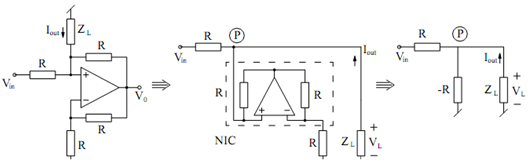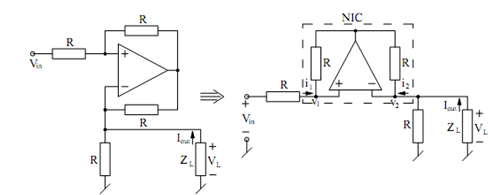## Voltage-Controlled Current Sources Assignment Help

Assignment Help: >> Basic Analog Circuits using Ideal Op-amps - Voltage-Controlled Current Sources

Voltage-Controlled Current Sources:

Consider the circuit illustrated in Figure (a). By judicious redrawing it may be rearranged as in Figure (b) where it is simple to recognize an NIC terminated into R realizing a -R at input port of NIC. As a result, we obtain the equivalent circuit of Figure (c). Analysis of this equivalent circuit gives

I out    =  VL /- R + (VL  - Vm  ) / R = - Vm  /R(a)                                                         (b)                                                       (c)

Figure: Realization of Voltage-controlled Current Sources

Therefore, the circuit of Figure (a) is an inverting VCCS.

A slightly different arrangement giving a non-inverting VCCS is illustrated in Figure (a).(a)                                                            (b)

Figure: A Non-inverting VCCS using an Op-amp

With some re-arrangement the circuit may be redrawn as in Figure (b) where once again, we may identify an NIC. For the NIC, we have

V1 = V2  = VL

also as the two resistors around the NIC are identical, we have  i1 = i2. But

i  = (Vm  - V1 ) /R and i2  = Iout - (V2 /R) = iout  - V1/R . From these equations, it follows that

(Vm  - V1  )/R = iout  - V1 /R which leads to

Iout  =+ Vm /R#### Assured A++ Grade

Get guaranteed satisfaction & time on delivery in every assignment order you paid with us! We ensure premium quality solution document along with free turntin report!

All rights reserved! Copyrights ©2019-2020 ExpertsMind IT Educational Pvt Ltd